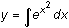Module 22 - Power Series Introduction | Lesson 1 | Lesson 2 | Lesson 3 | Self-Test Self Test Use the taylor command to find the fifth-order Maclaurin polynomial for y = tan-1 x. Graph y = tan-1 x with a "thick" graphing style. In the same window graph the 5th-, 9th- and 13th- order Maclaurin polynomials for this function. Estimate the interval of convergence for the series based on your graph. Use the taylor( command to find the second-order Taylor polynomial centered at 2 for y = ex. Use the taylor( command to find the fourth-order Maclaurin polynomial for y = ex2. Take the derivative of this polynomial. Use these results to predict the third-order Maclaurin polynomial for y = 2xex2. Verify your prediction by using the taylor( command to find the third-order Maclaurin polynomial for y = 2xex2. Use the taylor( command to find the fourth-order Maclaurin polynomial for y = ex2. Take the integral of this polynomial and use the result to predict the fifth-order Maclaurin polynomial for. Click here to check your answers. < Back ©Copyright 2007 All rights reserved. | Trademarks | Privacy Policy | Link Policy